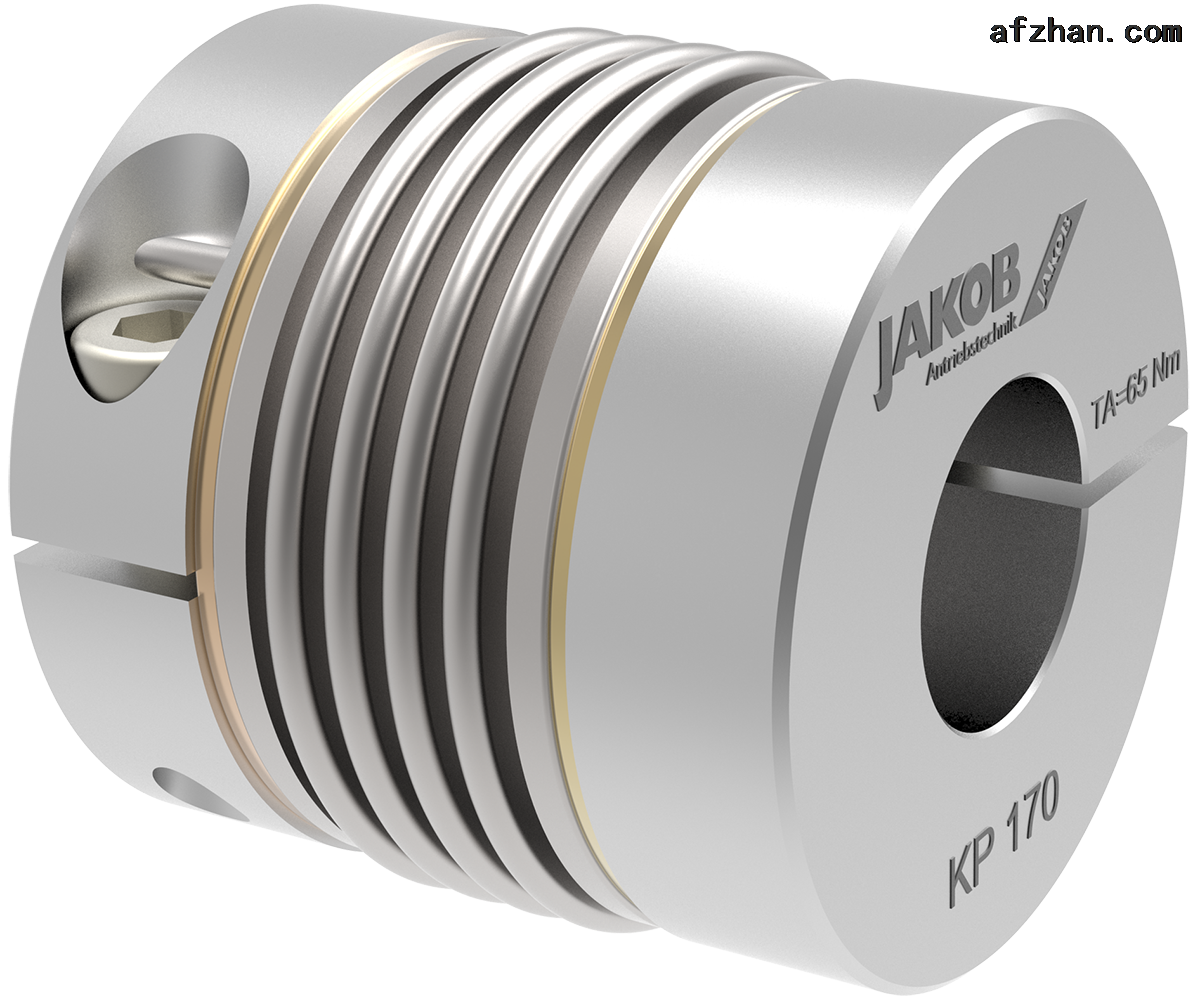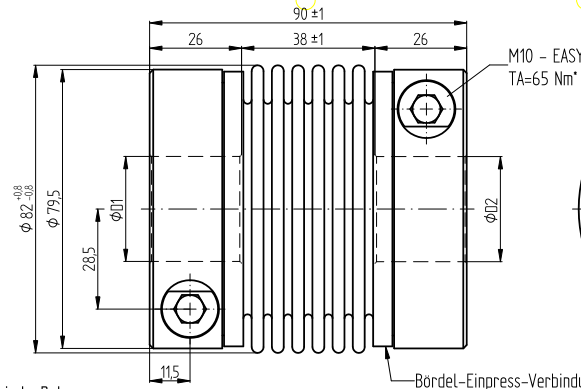JAKOB KM係列金屬波紋管聯軸器特點

JAKOB KM係列金屬波紋管聯軸器特點

6個波紋管--高性價比的標準波紋管係列
-扭矩範圍：20-1.300牛米。
-軸的直徑：8-85毫米

JAKOB KM係列金屬波紋管聯軸器特點

6個波紋管--高性價比的標準波紋管係列

-扭矩範圍：20-1.300牛米。

-軸的直徑：8-85毫米JAKOB金屬波紋管聯軸器係列KM產品型號及特點。

1JAKOB金屬波紋管聯軸器KM20

Øa=56c=19f=M6g=30h=8L=70D1=30G6D2=35H6

D1/2最小/最大=Ø8/Ø32mm2JAKOB金屬波紋管聯軸器KM60

Øa=66c=22f=M8g=33h=9L=77D1=28G6D2=42H6

D1/2最小/最大=Ø13/Ø35mmPoint: MB - 064 07711

3JAKOB金屬波紋管聯軸器KM80

Øa=82c=28.5f=M10g=38h=11.5L=90D1=30G6D2=42H6

D1/2最小/最大=Ø16/Ø43mmJAKOB金屬波紋管聯軸器係列KM

6個波紋管--高性價比的標準波紋管係列

-扭矩範圍：20-1.300牛米。

-軸的直徑：8-85毫米

JAKOB KM係列金屬波紋管聯軸器特點

JAKOB金屬波紋管聯軸器KM20Øa=56c=19f=M6g=30h=8L=70D1=30G6D2=35H6

JAKOB金屬波紋管聯軸器KM60Øa=66c=22f=M8g=33h=9L=77D1=28G6D2=42H6

JAKOB金屬波紋管聯軸器KM80Øa=82c=28,5f=M10g=38h=11,5L=90D1=30G6D2=42H6

留言框

• 驗證碼：請輸入計算結果（填寫阿拉伯數字），如：三加四=7010-64714988-173

[關閉]